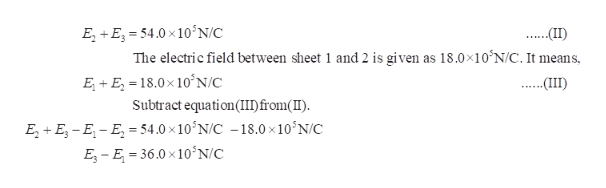Question
169 views

Figure (a) shows three plastic sheets that are large, parallel, and uniformly charged. Figure (b) gives the component of the net electric field along an x axis through the sheets. The scale of the vertical axis is set by Es = 9.0 × 105 N/C. What is the ratio of the charge density on sheet 3 to that on sheet 2?

check_circle

star
star
star
star
star
1 Rating
Step 1

From the figure (b), it can be seen that the net electric field at right side of sheet 3 is zero. For this to happen, there are three possibilities.

Step 2

The electric field between sheet 2 and 3 is given as 54.0×105 N/C. It means,help_outlineImage TranscriptioncloseE+E 54.0x10'N/C The electric field between sheet 1 and 2 is given as 18.0x10^N/C. It means E+E 18.0x 10N/C Subtract equation (II)from() E2+E-E-E 54.0 x 10'N/C -18.0 x 10 N/C E-E 36.0x10'N/C fullscreen
Step 3

Use the above relation ...

### Want to see the full answer?

See Solution

#### Want to see this answer and more?

Solutions are written by subject experts who are available 24/7. Questions are typically answered within 1 hour.*

See Solution
*Response times may vary by subject and question.
Tagged in

### Physics